Test Description

## 20 Questions MCQ Test Mathematics (Maths) Class 8 | Test: Understanding Quadrilaterals- 2

Test: Understanding Quadrilaterals- 2 for Class 8 2023 is part of Mathematics (Maths) Class 8 preparation. The Test: Understanding Quadrilaterals- 2 questions and answers have been prepared according to the Class 8 exam syllabus.The Test: Understanding Quadrilaterals- 2 MCQs are made for Class 8 2023 Exam. Find important definitions, questions, notes, meanings, examples, exercises, MCQs and online tests for Test: Understanding Quadrilaterals- 2 below.
Solutions of Test: Understanding Quadrilaterals- 2 questions in English are available as part of our Mathematics (Maths) Class 8 for Class 8 & Test: Understanding Quadrilaterals- 2 solutions in Hindi for Mathematics (Maths) Class 8 course. Download more important topics, notes, lectures and mock test series for Class 8 Exam by signing up for free. Attempt Test: Understanding Quadrilaterals- 2 | 20 questions in 20 minutes | Mock test for Class 8 preparation | Free important questions MCQ to study Mathematics (Maths) Class 8 for Class 8 Exam | Download free PDF with solutions
 1 Crore+ students have signed up on EduRev. Have you?
Test: Understanding Quadrilaterals- 2 - Question 1

### Which of the following is true for the adjacent angles of a parallelogram?

Detailed Solution for Test: Understanding Quadrilaterals- 2 - Question 1 Yes off course see 50+y =180 (co-interior angle) y =180-150 y =130
Test: Understanding Quadrilaterals- 2 - Question 2

### Find the value of the unknown z.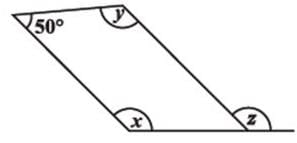Test: Understanding Quadrilaterals- 2 - Question 3

### Which of the following quadilaterals has two pairs of adjacent sides equal and diagonals intersecting at right angles?

Detailed Solution for Test: Understanding Quadrilaterals- 2 - Question 3

Rhombus, is a quadrilaterals has two pairs of adjacent sides equal and diagonals intersecting at right angles.

Test: Understanding Quadrilaterals- 2 - Question 4

RICE is a rhombus. Find x.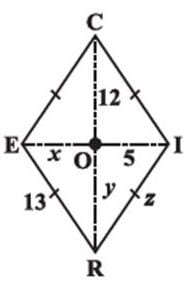Detailed Solution for Test: Understanding Quadrilaterals- 2 - Question 4

Given a rhombus RICE where
RE = 13, Ol = 5 OC = 12
In a rhombus,
Diagonals bisect each other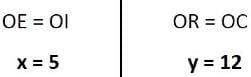Also,
All sides of a rhombus are equal.
RI = RE
x = 5
x = 5, y = 12 and z =13

Test: Understanding Quadrilaterals- 2 - Question 5

RICE is a rhombus. Find z.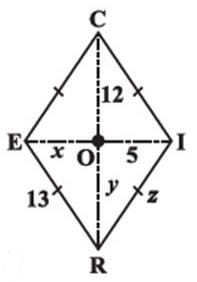Detailed Solution for Test: Understanding Quadrilaterals- 2 - Question 5

Given a rhombus RICE where
RE = 13, Ol = 5 OC = 12
In a rhombus,
Diagonals bisect each otherAlso,
All sides of a rhombus are equal.
RI = RE
z = 13
x = 5, y = 12 and z =13

Test: Understanding Quadrilaterals- 2 - Question 6

State the name of a regular polygon of 6 sides.

Test: Understanding Quadrilaterals- 2 - Question 7

Find the measure of each exterior angle of a regular polygon of 9 sides.

Detailed Solution for Test: Understanding Quadrilaterals- 2 - Question 7
Sum of all exterior angles of the given polygon = 360degree
Each exterior angle of a regular polygon has the same measure.
Thus, measure of each exterior angle of a regular polygon of 9 sides=360/9= 40degree.
Test: Understanding Quadrilaterals- 2 - Question 8

The sides of a pentagon are produced in order. Which of the following is the sum of its exterior angles?

Test: Understanding Quadrilaterals- 2 - Question 9

How many diagonals does a rectangle have?

Test: Understanding Quadrilaterals- 2 - Question 10

ABCD is a quadrilateral. If AC and BD bisect each other, what is ABCD?

Detailed Solution for Test: Understanding Quadrilaterals- 2 - Question 10 Sum of all angles of a quadrilateral is 360 degree . so we will add all the angles of quadrilateral and subtract it from 360 to get x. there is a linear pair on left side and exterior angle is 90 hence the other angle will be 90 by linear pair property. hence 70+60+90+x=360 220+x=360 x=360-220 x=140 hence C is the right answer.
Test: Understanding Quadrilaterals- 2 - Question 11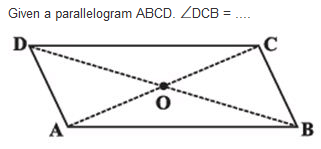Detailed Solution for Test: Understanding Quadrilaterals- 2 - Question 11

Test: Understanding Quadrilaterals- 2 - Question 12

A _______ is both ‘equiangular’ and ‘equilateral’.

Detailed Solution for Test: Understanding Quadrilaterals- 2 - Question 12 A regular polygon is eqiangular and equilateral as even the sides of such a polygon are equal. To verify u can use properties of a polygon(quadrilateral is the easiest).
Test: Understanding Quadrilaterals- 2 - Question 13

A _________ has all the properties of a parallelogram and also that of a kite.

Test: Understanding Quadrilaterals- 2 - Question 14

Find the value of the unknown y.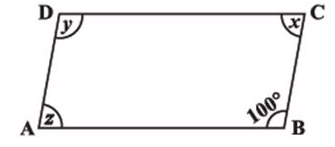Test: Understanding Quadrilaterals- 2 - Question 15

A simple closed curve made up of only line segments is called a ________.

Test: Understanding Quadrilaterals- 2 - Question 16

A _________ is a quadrilateral whose opposite sides are parallel.

Test: Understanding Quadrilaterals- 2 - Question 17

Find the value of the unknown x.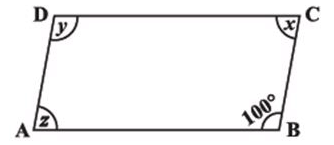Detailed Solution for Test: Understanding Quadrilaterals- 2 - Question 17

We have sum of adjacent angles equal to 180, so x+100=180x=80

Test: Understanding Quadrilaterals- 2 - Question 18

RENT is a rectangle. Its diagonals meet at O. Find x, if OR = 2x + 4 and OT = 3x + 1.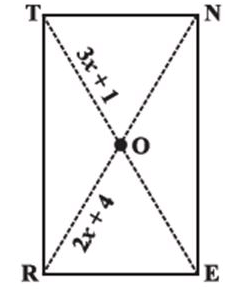Detailed Solution for Test: Understanding Quadrilaterals- 2 - Question 18

2x+4 = 3x+1 gives x = 3

Test: Understanding Quadrilaterals- 2 - Question 19

How many diagonals does a triangle have?

Detailed Solution for Test: Understanding Quadrilaterals- 2 - Question 19

A triangle has zero diagonals.Diagonals must be created across vertices in a polygon, but the vertices must not be adjacent to one another. A triangle has only adjacent vertices.

Test: Understanding Quadrilaterals- 2 - Question 20

Find the angle measure x in the following figure: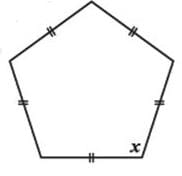Detailed Solution for Test: Understanding Quadrilaterals- 2 - Question 20

We have angle sum of pentagon=(5-2)*180 =540. Since it is a regular pentagon all angles are equal. So the sum is 5x. So each angle measures  x = 540/5 = 108º

## Mathematics (Maths) Class 8

193 videos|360 docs|48 tests
 Use Code STAYHOME200 and get INR 200 additional OFF Use Coupon Code